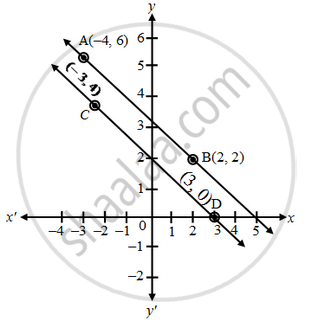Share

# Show graphically that the system of equations 2x + 3y = 10, 4x + 6y = 12 has no solution - CBSE Class 10 - Mathematics

ConceptGraphical Method of Solution of a Pair of Linear Equations

#### Question

Show graphically that the system of equations 2x + 3y = 10, 4x + 6y = 12 has no solution

#### Solution

The given equations are

2x + 3y = 10 ⇒ y = \frac { 10-2x }{ 3 }

 x -4 2 y 6 2 Points A B

4x + 6y = 12     ⇒      y =   \frac { 12-4x }{ 6 }

 x -3 3 y 4 0 Points C D

Plot the points A (–4, 6), B(2, 2) and join them to form a line AB. Similarly, plot the points C(–3, 4), D(3, 0) and join them to get a line CDClearly, the graphs of the given equations are parallel lines. As they have no common point, there is no common solution. Hence, the given system of equations has no solution.

Is there an error in this question or solution?
Solution Show graphically that the system of equations 2x + 3y = 10, 4x + 6y = 12 has no solution Concept: Graphical Method of Solution of a Pair of Linear Equations.
S